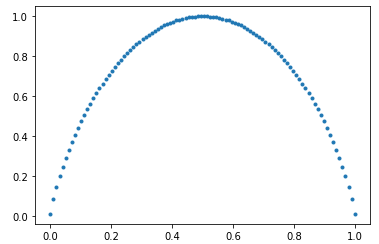# Kullback-Leibler Divergence

• Last Updated : 22 Jan, 2021

Entropy: Entropy is a way of measuring the uncertainty/randomness of a random variable XIn other words, entropy measures the amount of information in a random variable. It is normally measured in bits.

Joint Entropy: The joint Entropy of a pair of discrete random variables X, Y ~ p (x, y) is the amount of information needed on average to specify both their values.Conditional Entropy: The conditional entropy of a random variable Y given another X expresses how much extra information one still needs to supply on average to communicate Y given that the other party knows X.Example:

Calculate the Entropy of Fair coin:Here, the entropy of fair coin is maximum i.e 1. As the biasness of the coin increases the information/entropy decreases. Below is the plot of Entropy vs Biasness, the curve will look as follows:Biasness of Coin vs Entropy

Cross Entropy: Cross-entropy is a measure of the difference between two probability distributions (p and q) for a given random variable or set of events. In other words, Cross-entropy is the average number of bits needed to encode data from a source of distribution p when we use model q.

Cross-entropy can be defined as:Kullback-Leibler Divergence: KL divergence is the measure of the relative difference between two probability distributions for a given random variable or set of events. KL divergence is also known as Relative Entropy. It can be calculated by the following formula:The difference between Cross-Entropy and KL-divergence is that Cross-Entropy calculates the total distributions required to represent an event from the distribution q instead of p, while KL-divergence represents the extra amount of bit required to represent an event from the distribution q instead of p.

Properties of KL-divergence:

• D(p || q) is always greater than or equal to 0.

• D(p || q) is not equal to D(q || p).  The KL-divergence is not communicative.

• If p=q, then D(p || q) is 0.

Example and Implementation:

Suppose there are two boxes that contain 4 types of balls (green, blue, red, yellow). A ball is drawn from the box randomly having the given probabilities. Our task is to calculate the difference of distributions of two boxes i.e KL- divergence.

Code: Python code implementation to solve this problem.

 # box =[P(green),P(blue),P(red),P(yellow)]  box_1 = [0.25, 0.33, 0.23, 0.19]  box_2 = [0.21, 0.21, 0.32, 0.26]     import numpy as np  from scipy.special import rel_entr     def kl_divergence(a, b):      return sum(a[i] * np.log(a[i]/b[i]) for i in range(len(a)))       print('KL-divergence(box_1 || box_2): %.3f ' % kl_divergence(box_1,box_2))  print('KL-divergence(box_2 || box_1): %.3f ' % kl_divergence(box_2,box_1))     # D( p || p) =0  print('KL-divergence(box_1 || box_1): %.3f ' % kl_divergence(box_1,box_1))     print("Using Scipy rel_entr function")  box_1 = np.array(box_1)  box_2 = np.array(box_2)     print('KL-divergence(box_1 || box_2): %.3f ' % sum(rel_entr(box_1,box_2)))  print('KL-divergence(box_2 || box_1): %.3f ' % sum(rel_entr(box_2,box_1)))  print('KL-divergence(box_1 || box_1): %.3f ' % sum(rel_entr(box_1,box_1)))

Output:

KL-divergence(box_1 || box_2): 0.057
KL-divergence(box_2 || box_1): 0.056
KL-divergence(box_1 || box_1): 0.000
Using Scipy rel_entr function
KL-divergence(box_1 || box_2): 0.057
KL-divergence(box_2 || box_1): 0.056
KL-divergence(box_1 || box_1): 0.000 

Applications of KL-divergence:

Entropy and KL-divergence have many useful applications particularly in data science and compression.

• Entropy can be used in data preprocessing steps such as feature selections. For Example, If we want to classify the different NLP docs based on their topics, then we can check for the randomness of the different word appears in the doc. There is more chance of the word “computer” appears in technology-related docs but the same cannot be said for the word “the”
• The Entropy can also be used for text compression and quantifying the compression. The data which contains some pattern is easier to compress than the data which is more random.
• KL-divergence is also used in many NLP and computer vision models such as in Variational Auto Encoder to compare the original image distribution and distribution of images generated from the encoded distribution.

My Personal Notes arrow_drop_up
Recommended Articles
Page :